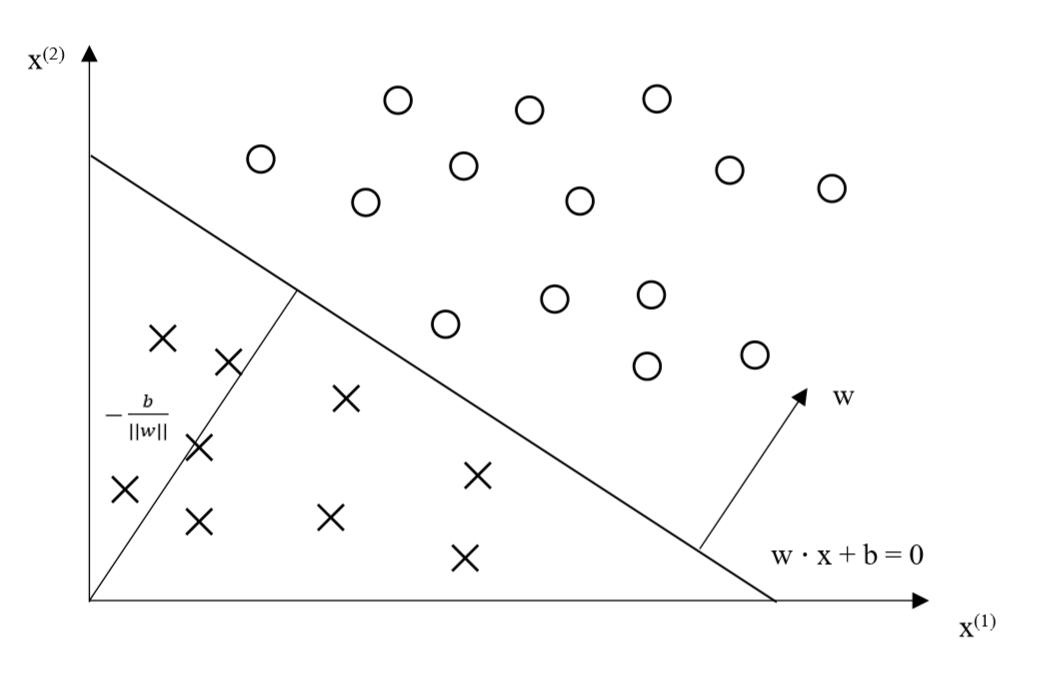# 机器学习：感知机模型

(在苹果系统下，如果文章中的图片不能正常显示，请升级Safari浏览器到最新版本，或者使用Chrome、Firefox浏览器打开。)

# 模型介绍

$$f(x)=sign(w \cdot x+b) \tag{1}$$

$$sign(x)= \left\{\begin{array}{l}+1 , x \ge 0\\-1, x＜0\end{array}\right. \tag{2}$$

$$w \cdot x+b=0 \tag{3}$$# 学习策略

$$\frac{1}{||w||} | w \cdot x_0 + b | \tag{4}$$

$$-y_i (w \cdot x_0 +b)>0 \tag{5}$$

$$\frac{-1}{||w||} y_i ( w \cdot x_i + b ) \tag{6}$$

$$\frac{-1}{||w||} \sum_{x_i \in M} {y_i (w \cdot x_i +b)} \tag{7}$$

$$T={(x_1,y_1),(x_2,y_2),…,(x_N,y_N)} \tag{8}$$

$$L(w,b)=-\sum_{x_i \in M}{y_i(w \cdot x_i+b)} \tag{9}$$

# 学习算法

$$T={(x_1,y_1),(x_2,y_2),…,(x_N,y_N)} \tag{10}$$

$$min_{w,b} L (w,b)=-\sum_{x_i \in M}{y_i (w \cdot x_i + b)} \tag{11}$$

$$\bigtriangledown_w L(w,b)=-\sum_{x_i \in M}{y_i x_i} \tag{12}$$ $$\bigtriangledown_b L(w,b)=-\sum_{x_i \in M}{y_i} \tag{13}$$

$$w ← w+ η y_i x_i \tag{14}$$ $$b ← b + η y_i \tag{15}$$

(1)选取初值$$w_0, b_0$$

(2)在训练集中选取数据$$(x_i, y_i)$$

(3)如果$$y_i(w * x_i + b) \le 0$$

$$w ← w+ η y_i x_i$$

$$b ← b + η y_i$$

(4)转至(2)，直到训练集中没有误分类点.

# 参考资料

 李航. 统计学习方法[M]. 北京: 清华大学出版社, 2012, p25-36.

 周志华. 机器学习[M]. 北京: 清华大学出版社, 2016, p98-101.

 版权声明本博客的文章除特别说明外均为原创，本人版权所有。欢迎转载，转载请注明作者及来源链接，谢谢。本文地址: https://blog.ailemon.net/2019/10/09/machine-learning-perceptron-model/ All articles are under Attribution-NonCommercial-ShareAlike 4.0